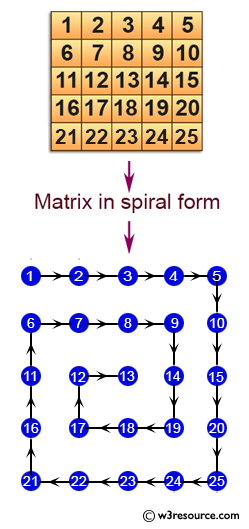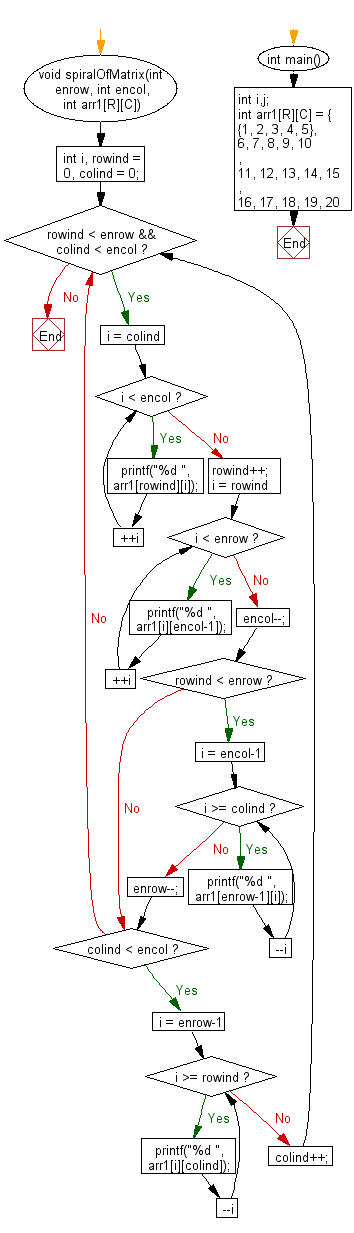﻿ C exercises: Print a matrix in spiral form - w3resource# C Exercises: Print a matrix in spiral form

## C Array: Exercise-50 with Solution

Write a program in C to print a matrix in spiral form.

Pictorial Presentation:Sample Solution:

C Code:

``````#include <stdio.h>
#define R 4
#define C 5
void spiralOfMatrix(int enrow, int encol, int arr1[R][C])
{
int i, rowind = 0, colind = 0;
while (rowind < enrow && colind < encol)
{
for (i = colind; i < encol; ++i)
{
printf("%d ", arr1[rowind][i]);
}
rowind++;
for (i = rowind; i < enrow; ++i)
{
printf("%d ", arr1[i][encol-1]);
}
encol--;
if ( rowind < enrow)
{
for (i = encol-1; i >= colind; --i)
{
printf("%d ", arr1[enrow-1][i]);
}
enrow--;
}
if (colind < encol)
{
for (i = enrow-1; i >= rowind; --i)
{
printf("%d ", arr1[i][colind]);
}
colind++;
}
}
}
int main()
{
int i,j;
int arr1[R][C] = { {1,  2,  3,  4,  5},
{6, 7,  8,  9,  10},
{11, 12, 13, 14, 15},
{16, 17, 18, 19, 20}
};
//------------- print original array ------------------
printf("The given array in matrix form is :  \n");
for(i = 0; i < R; i++)
{
for (j=0;j<C;j++)
{
printf("%d  ", arr1[i][j]);
}
printf("\n");
}
//------------------------------------------------------
printf("The spiral form of above matrix is: \n");
spiralOfMatrix(R, C, arr1);
return 0;
}```
```

Sample Output:

```The given array in matrix form is :
1  2  3  4  5
6  7  8  9  10
11  12  13  14  15
16  17  18  19  20
The spiral form of above matrix is:
1 2 3 4 5 10 15 20 19 18 17 16 11 6 7 8 9 14 13 12
```

Flowchart:C Programming Code Editor:

Improve this sample solution and post your code through Disqus.

What is the difficulty level of this exercise?

﻿

## C Programming: Tips of the Day

Where is the C auto keyword used?

auto is a modifier like static. It defines the storage class of a variable. However, since the default for local variables is auto, you don't normally need to manually specify it.

Ref : https://bit.ly/3yzwC9r